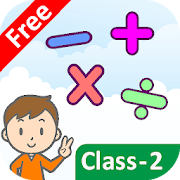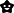Class 2 Math Complete SyllabusEveryoneFamily Friendly
3
Full Class 2 Math App with interactive games and sounds that help your kids to learn Numbers, Addition, Subtraction, Multiplication, Division, Fraction, Shapes, Lines, Segments, Length, Time, Clock and much more in a fun manner.
This App is prepared as per CBSE, NCERT and State Board Grade 2 Math course.

2nd Standard Math Complete syllabus includes

Numbers for Class 2

1. Give Number's Name
2. Give Numeral
3. Biggest Number
4. Smallest Number
5. Greater / Smaller / Equal
6. After Number
7. Between Number
8. Before Number
9. Skip Counting
10. Ascending Order
11. Descending Order
12. Underline Digit
13. Place Value
14. Number on Abacus
15. How Many HTO?
16. Expanded Form
17. Short Form
18. Odd Number
19. Even Number

1. Introduction
2. 2-3 Digit Addition (Without Carryforward )
3. 2-3 Digit Addition (With Carryforward)
4. 2 Digit 3 Number Addition
5. 3 Digit 3 Number Addition

Subtraction for Class 2

1. Introduction.
2. 2-3 Digit Subtraction. (Without borrowing)
3. 2-3 Digit Subtraction. (With borrowing)
4. 2 Digit 3 Number Subtraction.
5. 3 Digit 3 Number Subtraction.
6. Story Subtraction.

Multiplication for Class 2

1. Introduction.
2. 1 Digit Multiplication.
3. 2 Digit Multiplication.
4. 3 Digit Multiplication.
5. Story Multiplication

Division for Class 2

1. Introduction.
2. 1 Digit Division
3. 2 Digit Division
4. Long Division (find quotient)
5. Long Division (find reminder)
6. Story Division

Fractions for Class 2

1. Introduction.
3. Fraction for Given Number.
4. Fraction of Collection

Shapes for Class 2

1. The shape of an Object.
2. Same Shape & Same Size
3. Roll or Slide
4. Slides and Corners
5. Fill in the Blanks

Point, Lines, and Line Segments for Class 2

1. Introduction.
2. Curved and Straight Lines.
3. How many Lines?
4. Point & Lines Segment.

Length for Class 2

1. Introduction to Length
2. Meter to Centimeters
3. 'm' or 'cm'
5. Length Subtraction
6. Introduction to Weight
7. Gram to Kilogram
8. 'g' or 'kg'
10. Weight Subtraction

Capacity for Class 2

1. Introduction to Capacity
2. Liter to Mililitre
3. 'l' or 'ml'
5. Capacity Subtraction

Time, Day and Months for Grade 2

1. Introduction to Time and Clocks
2. Time on Clock
3. Fill in the blanks

Money

1. Price Tag
2. How Much Money?
3. Convert rupee to Paisa
4. Convert Paisa to rupee
6. Money Subtraction

Our 2nd Standard Math App is a complete guide to learning numbers, addition, division, fractions and much more in a fun way for your kids.

Let's make learning fun!

For more free educational apps visit https://thegreatkids.com/
Collapse

Review Policy
5.0
3 total
5
4
3
2
1

What's New

Class 2 Maths 1500+ examples for Addition, subtraction, Multiplication, Division as per CBSE, NCERT and State Board Syllabus.
Collapse

Updated
June 10, 2019
Size
21M
Installs
100+
Current Version
4.0
Requires Android
4.0 and up
Content Rating
Everyone
Permissions
Offered By